# Fluids physical properties

## density, viscosity, pressure# Need a helping hand?If you need a quick calculation, but you are not still familiar how to use the calculator, you can order calculation service from the calculator developer.

### Density of liquid and gas, compressibility factor. Gas pressure, temperature and density relation

The density is the mass of substance per unit of volume.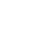where is: ρ - density; m - mass; V - volume.

The unit of density is kilogram per cubic meter [kg/m³] in SI system of units and used symbol is ρ . Also, other units are used for density like gram per cubic centimeter [g/cm³], or gram per milliliter [g/ml] which are both equal to 1000 kilograms per cubic meter [kg/m³].

For perfect gas relation between pressure, density and temperature is expressed using the equation of state: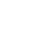where is: p - pressure; ρ - density; R - gas constant; T - absolute temperature.

For real gas modified equation of state is used with compressibility factor included: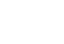where is: p - pressure; ρ - density; T - absolute temperature; Z - compressibility factor; R - gas constant.

Compressibility factor is function of pressure and temperature. Compressibility factor can be calculated using Berthelot equation: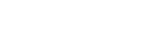where is: pr - reduced pressure; Tr - reduced temperature; Z - compressibility factor.

which are calculated as follows: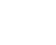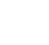where is: pc - critical pressure; Tc - critical temperature.

### Specific volume and density

Specific volume is the volume of substance per unit of mass, and is reciprocal to density. The unit for specific volume is cubic meter per kilogram [m3/kg] and used symbol is v. Other commonly used units for specific volume are liter per kilogram [l/kg] or cubic decimeter per kilogram [dm3/kg] which are equal to 0.001 m3/kg.

Density and specific volume of liquids are changing with the change of temperature, and if high pressures are being considered, density and specific volume will change as well.

When gases are considered, the density and specific volume are changing greatly with the change of pressure and temperature.

### Specific gravity of water and oil

Specific gravity (or relative density) is a relative measure of density and is ratio between the density of fluid at a specified temperature to the water density at some standard temperature. The temperatures are usually the same and value of 60OF/60OF (15.6OC/15.6OC) is commonly used. For measuring of specific gravity a hydrometer can be used, and two scales are common - API scale, used for oils and Baume scales with two kinds in use - one for liquids heavier than water and one for liquids lighter than water.

For conversion between API and Baume scales on one side and specific gravity on the other side:

For oils specific gravity SG(60OF/60OF):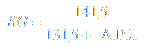For liquids lighter than water SG(60OF/60OF):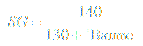For liquids heavier than water SG(60OF/60OF):As the density of water at 15OC is 999 kg/m3, density of oil or other liquid can be calculated as: water density x SG(60OF/60OF).

Use specific gravity calculator for conversion between API degrees, Baume degrees and specific gravity.

The specific gravity of gases is defined as weight ratio between molecular weight of the gas and the air, and as ratio between individual gas constant of air and gas being considered.

### Density of fluid mixture for known volume or weight ratio of component in mixture

If the fluid being considered is the mixture of several components, physical properties such as density are calculated based on the property of each component with its volume or weight ratio used. One of fluids that is actually mixture of several components is liquefied petroleum gas or LPG. LPG is mixture of propane (C3H8), butane (C4H10) and other components. The density of such mixture is calculated as defined bellow.

Density of fluid mixture is calculated as: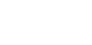where is: ρ MIX - density of fluid mixture; i - density of component i; ri - volume ratio of component i; gi - weight ratio of component i.

If volume of each component in the fluid mixture is known, than component volume ratio can be calculated as:where is: ri - volume ratio of component i; Vi - volume of component i; VMIX - volume of fluid mixture; n - total number of components.

If weight of each component in fluid mixture is known, than component weight ratio can be calculated as:where is: gi - weight ratio of component i; mi - weight of component i; mMIX - weight of fluid mixture; n - total number of components.

For known weight ratio, volume ratio of each component in fluid mixture is calculated: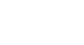For known volume ratio, weight ratio of each component in fluid mixture is calculated:Molar weight of the fluid mixture is: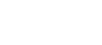where is: Mi - molar weight of component i; MMIX - molar weight of mixture; gi - weight ratio of component i; ri - volume ratio of component i.

These relations are used in calculator for LPG - liquefied petroleum gas flow rate and pipe diameter calculation.

### Density and partial pressure of component in gas mixture

If the mixture is consisting of gases then density of each component in the mixture can be calculated as: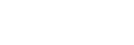Partial pressure of one component in the mixture of gases is: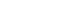where is: ρi - density of component i; pi - partial pressure of component i; pMIX - pressure of mixture; ri - volume ratio of component i; gi - mass ratio of component i; Ri - gas constant of component i; RMIX - gas constant mixture; T - absolute temperature of gas; Z - compressibility factor.

Gas constant for the mixture of gases can be calculated as: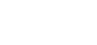where is: ri - volume ratio of component i; gi - mass ratio of component i; Ri - gas constant of component i; RMIX - gas constant mixture; Mi - molar mass of component i.

These relations are used in calculator for LPG - liquefied petroleum gas flow rate and pipe diameter calculation.

### Dynamic or absolute viscosity

For dynamic or absolute viscosity used unit in SI system is [Pas] - Pascal second, which may also be expressed as the [Ns/m2] or as the [kg/ms]. This unit has also been called the Poiseuille [Pl] in France, but it should be noted that it is not the same as the poise - [P] described below.

The Poise is the dynamic or absolute viscosity unit in the CGS system of units. The submultiple centipoise [cP], 0,01 P, is the unit most commonly used at present to express dynamic viscosity.

The relationship between Pascal second and centipoise is:

1 Pas = 1 Ns/m2=1 kg/(ms)=103 cP
1 cP = 10-3 Pas

The symbol used for dynamic or absolute viscosity is μ . The viscosity of water at temperature of 20 degrees C is very nearly 1 cP, or 0.001 Pas. Actually the viscosity of water at 20 degrees C is 1.002 cP.

Dynamic or absolute viscosity is changing with the change of temperature and pressure. With the temperature rise, viscosity of liquids is decreasing and viscosity of gases is increasing.

For temperature differences in the range of -20OC and 500OC, dynamic or absolute viscosity can be calculated as: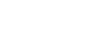where is: μT - dynamic or absolute viscosity at temperature T; μTO - reference dynamic or absolute viscosity at temperature T0; T, TO - temperature; m = 0.76 for air, 0.874 for methane, 0.866 for carbon-dioxide, 1.05 for ammonia;

Gas mixture dynamic or absolute viscosity can be calculated using (Herning-Zipperer):where is: ri - volume ratio of component i; μ - dynamic or absolute viscosity of mixture; μi - dynamic or absolute viscosity of component i; Mi - molar mass of component i; Tci - critical temperature of component i;

### Kinematic viscosity

The ratio of the dynamic viscosity to the density is kinematic viscosity:where is: ν - kinematic viscosity; μ - dynamic viscosity; ρ - density;

The unit for kinematic viscosity is the meter squared pre second [m2/s]. In CGS system of units, for kinematic viscosity is used stokes (St) and centistokes (cSt). The symbol used for kinematic viscosity is ν .

1 m2/s = 10.000 St
1 m2/s = 1.000.000 cSt
1 St = 100 cSt
ν [cSt] = μ [cP] / ρ [g/cm3]

### Pressure level definitions - absolute, barometric, gauge pressure and perfect vacuum

Although perfect vacuum cannot exist on the surface of the Earth, it makes convenient datum for the measurement of pressure.

Barometric pressure is the level of atmospheric pressure above perfect vacuum.

Standard atmospheric pressure is 101 325 Pa (1.013 25 bar, 14.6959 lbf/in2).

Gauge pressure is measured above atmospheric pressure.

Absolute pressure is measured with the reference to perfect vacuum as a base.

Vacuum is the depression of pressure below the atmospheric pressure level.

Vacuum is often represented by water or mercury column height in inches or mm in the sense of absolute pressure.

• Pipe flow theory pages
• Fluid properties
Fluid density, specific volume, specific gravity, mixture density, viscosity, pressure levels
• Incompressible flow in pipe
Pipe diameter, Reynolds number, laminar/turbulent flow, Darcy formula, friction factor, dynamic/total pressure, heat energy
• Flow in valves and fittings
Resistance coefficient, flow coefficient, cavitation, chocked flow, flow offtake, gas regulator, natural gas control valves
• Compressible flow
Adiabatic, isothermal flow, fuel combustion, maximum flow, evaporation energy
Pipe flow calculators